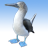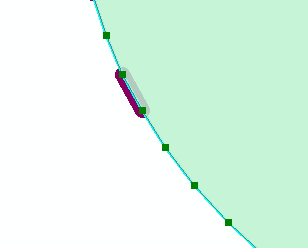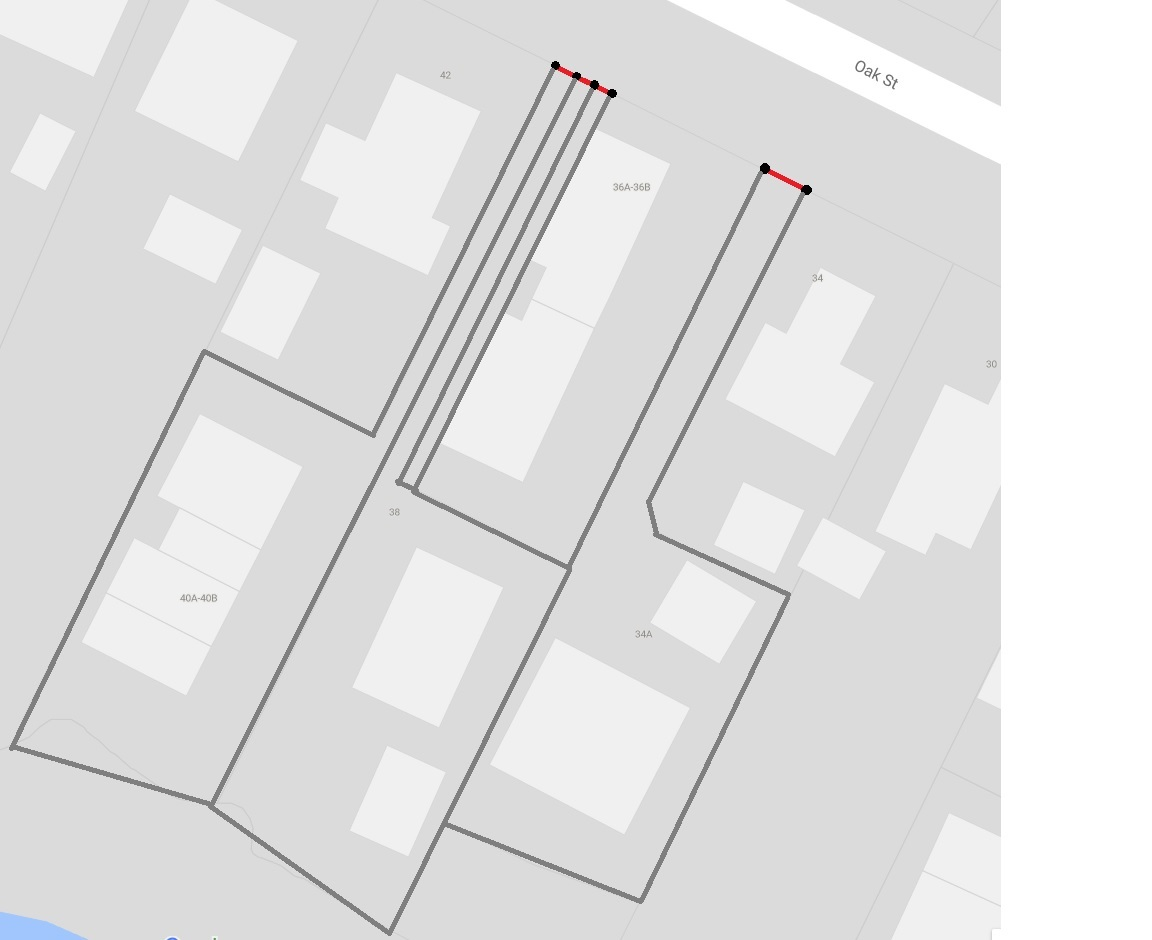# Finding the shortest edge length of polygons

1857
5
10-11-2017 02:55 PMNew Contributor II

I have 2,000+ polygons that I need to find the shortest perimeter edge for. Does anyone have any solutions? Thanks in advance!

Tags (3)
5 RepliesbyMVP Esteemed Contributor

There is no direct tool for that.  The process would entail scripting

• convert each polygon to a polyline
• devolve each polyline into its parts
• calculate the length of each segment, retaining the shortest length while cycling through the parts.

Concerns

• you will need to ensure that each polygon initially has extraneous vertices removed (Generalize, removing points on the perimeter that aren't part of a direction change.
• do you need the actual edge or do you need to get a measure of the shortest of the width or height of the minimum area bounding rectangle or the minimum bounding extent

Perhaps you could elaborate on the purpose for identifying the 'shortest polygon edge'by
Occasional Contributor III

Concerns

• you will need to ensure that each polygon initially has extraneous vertices removed (Generalize, removing points on the perimeter that aren't part of a direction change.

To add to this, a shape you see as a 'rectangle', may actually be composed of smaller edges that look like 4 edges when you zoom out far enough inside the GIS.  (e.g. the computer sees it as a 18 sided polygon, not a rectangle!) The generalise tool can help simplify the shape, but you need to enter tolerances that make sense with your particular data.

Simple example:

Actual Coords (5 sided shape to the computer):

1,1      1,2     2,2     2,1      2.02, 1.02

Human interpreted coords (Shape looks like a rectangle to me as the final vertex added nothing):

1,1      1,2     2,2     2,1

In this example, if I generalise the input data with a 0.05m tolerance, it would clean out the node thats not required as the 0.02 metre deviation has less effect on the shape of the polygon than the 0.05 tolerance.. and is therefore not required.byEsri Esteemed Contributor

I have used the code below in the past to find the longest edge of a polygon, but you could rewrite it to find the shortest edge. However, there could be some discussion on what an edge is or how this should be interpreted:

``def GetLargestLine(polygon, offset):    max_length = 0    prev_pnt = None    side = None    sr = polygon.spatialReference    polygon2 = polygon.generalize(0.1)    for part in polygon2:        for pnt in part:            # print pnt            if prev_pnt is None:                pass            else:                if pnt is None:                    pass                else:                    length = GetDist(pnt, prev_pnt)                    if length > max_length:                        max_length = length                        side = arcpy.Polyline(arcpy.Array([prev_pnt, pnt]), sr)            prev_pnt = pnt    length = side.length    side = side.segmentAlongLine(offset, length-offset, False)    return side, length‍‍‍‍‍‍‍‍‍‍‍‍‍‍‍‍‍‍‍‍‍‍‍‍``

This was used to generate solar panels on roof tops. See document here (Spanish content):byEsri Esteemed Contributor

Hust did a little test to see if it would work and I get a result with this code (it will add fields to the input featureclass, so it's better to run this on a copy of your data):

``def main():    # will change the input fc (adding fields!)    import arcpy    fc = r'C:\GeoNet\ShortestEdge\data.gdb\polygons'    generalize = 0.1 # change this value according to the tolerance in your data    fc_out = r'C:\GeoNet\ShortestEdge\data.gdb\lines_v01'    # output fields    fld_len1 = "ShortestLength1"    fld_from_pnt1 = "FromPoint1"    fld_to_pnt1 = "ToPoint1"    fld_len2 = "ShortestLength2"    fld_from_pnt2 = "FromPoint2"    fld_to_pnt2 = "ToPoint2"    # add fields    AddField(fc, fld_len1, "DOUBLE", None)    AddField(fc, fld_from_pnt1, "TEXT", 100)    AddField(fc, fld_to_pnt1, "TEXT", 100)    AddField(fc, fld_len2, "DOUBLE", None)    AddField(fc, fld_from_pnt2, "TEXT", 100)    AddField(fc, fld_to_pnt2, "TEXT", 100)    # update cursor    feats = []    flds = ('SHAPE@', fld_len1, fld_from_pnt1, fld_to_pnt1,             fld_len2, fld_from_pnt2, fld_to_pnt2)    with arcpy.da.UpdateCursor(fc, flds) as curs:        for row in curs:            polygon = row            length1, from_pnt1, to_pnt1, line1 = GetShortestEdgeData(polygon)            feats.append(line1)            polygon2 = polygon.generalize(generalize)            length2, from_pnt2, to_pnt2, line2 = GetShortestEdgeData(polygon2)            feats.append(line2)            curs.updateRow((polygon, length1, from_pnt1, to_pnt1, length2, from_pnt2, to_pnt2, ))    # write lines to output fc for visual reference    arcpy.CopyFeatures_management(feats, fc_out)def AddField(fc, fld_name, fld_type, fld_length):    if len(arcpy.ListFields(fc, fld_name)) == 0:        arcpy.AddField_management(fc, fld_name, fld_type, None, None, fld_length)def GetShortestEdgeData(polygon):    min_length = 99999    prev_pnt = None    side = None    sr = polygon.spatialReference    for part in polygon:        for pnt in part:            if prev_pnt is None:                pass            else:                if pnt is None:                    pass                else:                    length = GetDist(pnt, prev_pnt)                    if length < min_length:                        min_length = length                        line = arcpy.Polyline(arcpy.Array([prev_pnt, pnt]), sr)            prev_pnt = pnt    length = line.length    from_pnt = line.firstPoint    to_pnt = line.lastPoint    return length, str(from_pnt), str(to_pnt), linedef GetDist(pnt1, pnt2):    import math    return math.hypot(pnt2.X - pnt1.X, pnt2.Y - pnt1.Y)if __name__ == '__main__':    main()‍‍‍‍‍‍‍‍‍‍‍‍‍‍‍‍‍‍‍‍‍‍‍‍‍‍‍‍‍‍‍‍‍‍‍‍‍‍‍‍‍‍‍‍‍‍‍‍‍‍‍‍‍‍‍‍‍‍‍‍‍‍‍‍‍‍‍‍‍‍‍‍‍‍‍‍‍``

It will do this on the input polygon and a generalized polygon (set the variable generalize on line 5 with a proper value):It also creates an output featureclass with the shortest edges.New Contributor II